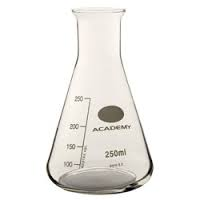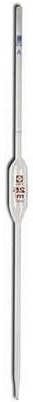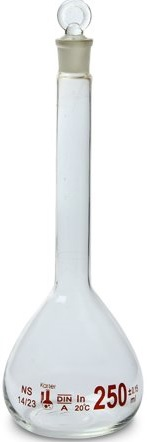Dilution of Solutions Techniques and Calculations (dilution equation: c1V1=c2V2)

Key Concepts

• Dilution means to reduce the concentration of a solution.
• A solution is produced when a solute dissolves in a solvent.

termdescriptionexample
solute:substance which dissolvessolid sodium chloride, NaCl(s)
solvent:substance which enables the solute to dissolveliquid water, H2O(l)
solution:prepared by dissolving a solute in a solventNaCl(aq) is NaCl(s) dissolved in H2O(l)

• Concentration refers to the amount of solute dissolved in a given volume of solution.

amount of solute volume of solution concentration molesmol litresL moles per litre (molarity)mol L-1 (mol/L or M)

concentration of a stock solution in mol L-1 = moles of solute ÷ volume of solution in litres

c1 = n1 ÷ V1
c1 = molarity of stock solution (concentration of stock solution in mol L-1)
n1 = moles of solute dissolved (in mol)
V1 = volume of stock solution (in L)

• A solution can be diluted by adding more solvent to the stock solution (the starting solution before dilution) in the same vessel.
The dilution equation (dilution formula or dilution expression) is:
c1V1 = c2V2

c1 = concentration of stock solution (before dilution) in mol L-1
V1 = volume of stock solution present before dilution in L
c2 = concentration of new dilute solution (after more solvent added) in mol L-1
V2 = total volume of the new dilute solution in L
Note: V2 > V1 and c2 < c1

 find calculation determine concentration of dilute solution c2 = c1V1 ÷ V2 determine volume of dilute solution V2 = c1V1 ÷ c2 determine concentration of stock solution before dilution c1 = c2V2 ÷ V1 determine volume of stock solution used (before dilution) V1 = c2V2 ÷ c1

• A solution can be diluted by using a pipette to transfer some of the stock solution to a volumetric flask and then adding solvent up to the mark:
The dilution equation (dilution formula or dilution expression) is:
c1V1 = c2V2

c1 = concentration of stock solution (before dilution) in mol L-1
V1 = volume of pipette used to transfer the stock solution in L
c2 = concentration of new dilute solution (after water added) in mol L-1
V2 = volume of volumetric flask used to prepare the dilute solution in L

 find calculation determine concentration of dilute solution c2 = c1V1 ÷ V2 determine volume of dilute solution V2 = c1V1 ÷ c2 determine concentration of stock solution c1 = c2V2 ÷ V1 determine volume of pipette used V1 = c2V2 ÷ c1

No ads = no money for us = no free stuff for you!

Dilution Technique

Dilution means to reduce the concentration of a solution.

A solution can be diluted by adding solvent to a given volume of stock solution.

In order to dilute a solution we need:

• A container of solution of known concentration.
This is the stock solution, the solution to be diluted.
• A pipette to remove a known volume of this solution from the container and transfer it to a new container such as a volumetric flask.
• A container of pure solvent, the same solvent used to make the original solution, such as water.
• A way of adding the pure solvent to the volumetric flask, for example a glass funnel and a pasteur pipette.

Step Description Calculation
1 The solution to be diluted will be in a vessel such as a volumetric flask. This solution is referred to as the stock solution.
In order to prevent contamination of this stock solution, place a small amount of it in a clean, dry, conical flask (erlenmeyer flask), swirl it around and then discard this solution appropriately.
DO NOT use water or any other solvent to rinse the flask because adding more solvent would dilute the solution and we would no longer know what the concentration of this solution is.
Stopper the conical flask when you are not using it to prevent contamination from the atmosphere.
Label it with the date, and the formula of the solution and its concentration, for example [NaCl(aq)] = 0.10 mol L-1
Record the concentration of this undiluted stock solution as c1 in mol L-1
c1 = [undiluted solution] mol L-12 You will need a clean, dry, pipette with a known volume.
This will be printed on the pipette, for example 10.00 mL, 25.00 mL, etc
Use the pipette filler (bulb) to draw up a small amount of stock solution from the conical flask, remove the pipette from the solution, swirl the solution in the pipette around to rinse it and then discard this solution suitably.
DO NOT use water or any other solvent to rinse the pipette because you would be diluting the solution and we would no longer know what the concentration of this solution is.
Place the rinsed pipette into the stock solution in the conical flask and draw up the solution until it is past the level marked on the pipette.
Remove the pipette from the stock solution.
Suspended the pipette within the confines of a beaker.
Allow the solution to escape from the pipette drop by drop until the bottom of the meniscus (when viewed at eye level) sits just on the level marked on the pipette.
You need to record the volume of this undiluted, stock solution, held in the pipette.
It is a good idea to convert this volume in milliltres (mL) to a volume in litres (L) if your concentration is mol L-1 (molarity):
volume in L = volume in mL ÷ 1000
Record the volume of this pipette as V1, for example, V(NaCl)1 = 10.00 mL = 10.00/1000 = 0.0100 L
V1 = [volume of undiluted solution in pipette] L
V1 = V(undiluted solution) L3 Position the pipette so that the tip of the pipette meets the inside neck of the clean volumetric flask at an angle.
Allow the solution in the pipette to escape and run down the neck of the volumetric flask into the body of the flask.
Pipettes are usually designed to deliver a known volume, this means that the small drop of solution remaining in the tip of the pipette is supposed to be there, so don't try to blow it out.
We now have a known volume of the undiluted stock solution, with a known concentration in this volumetric flask.
Calculate the moles of solute, n(solute), contained in this volumetric flask.
moles of solute (mol) = concentration of solution (mol L-1) × volume of solution delivered from pipette (L)
n(solute) = c1 × V1
Record the moles of solute, n(solute) in mol.
n(solute) = c1 × V1 mol

4 Place a glass funnel in the neck of the volumetric flask.
As you pour your solvent, for example water, into the funnel, leave a small air gap between the stem of the funnel and the neck of the volumetric flask so that the solvent flows freely.
When the solvent reaches the base of the neck of the volumetric flask, stop pouring and allow any solvent remaining in the funnel to flow through.
Slowly, and carefully, add more solvent until the bottom of the meniscus is about 1 cm from the level marked on the neck of the volumetric flask when viewed at eye level.
Use a clean pasteur pipette that has been rinsed with the solvent to add more solvent drop-by-drop to the volumetric flask until the bottom of the meniscus sits exactly on the level marked on the neck of the flask when viewed at eye level.
Record the volume of the diluted solution in this volumetric flask as V2 in mL
Convert the volume in millilitres to a volume in litres dividing by 1000.
Record this volume as V2 L.
V2 = [dilute solution] L5 Calculate the concentration of the dilute solution, c2:
concentration of dilute solution = moles of solute ÷ volume of dilute solution
molarity of dilute solution (mol L-1) = moles solute (mol) ÷ volume of dilute solution (L)
c2 = n(solute) ÷ V2
Affix a label to the volumetric flask which states the date the solution was made, its formula and concentration.
c2 = [dilute solution] mol L-1
c2 = n(solute) ÷ V2 mol L-1

Do you know this?

Play the game now!

The Dilution Equation (dilution formula, or, dilution expression)

We can summarise the process of diluting a stock solution as:

 undiluted solution → pipette → volumetric flask → add solvent → dilute solution c(undiluted solution) mol L-1 V(pipette) in mLV(pipette) in mL ÷ 1000 = V(pipette) L n(solute) = c(undiluted solution) × V(pipette in L) mol V(dilute solution) in mL V(dilute solution in mL) ÷ 1000 = V(dilute solution in L) c(dilue solution) = n(solute) ÷ V(dilute solution in L) c1in mol L-1 V1 in L n(solute) = c1 × V1 in mol V2 in L c2 = n(solute) ÷ V2 in mol L-1 or c2 = (c1 × V1) ÷ V2 in mol L-1

So we now have an equation (expression or formula) to calculate the concentration of a solution after it has been diluted:

 c2 = c1 × V1V2

 c1 = concentration of undiluted solution in mol L-1 V1 = volume of stock solution added to the volumetric flask = volume of pipette used in L c2 = concentration of the dilute solution in mol L-1 V2 = volume of volumetric flask holding the dilute solution in L

If we rearrange this equation (expression or formula) by multiplying both sides of the equation by V2 we get:

c1V1 = c2V2

which simply means that the moles of solute transferred by pipette and placed in the volumetric flask (n1) equals the moles of solute present in the volumetric flask after more solvent was added during the dilution (n2).

 moles solute transferred by pipette to volumetric flask = moles solute in volumetric flask after solvent added (dilute solution) c1V1 = c2V2 n1 = n2

This equation (formula or expression) is very useful because we can rearrange it find:

• c1 the concentration of the undiluted stock solution:
c1 = c2V2 ÷ V1
• V1 the volume of pipette required to transfer the undiluted solution to the volumetric flask for dilution:
V1 = c2V2 ÷ c1
• V2 the volume of volumetric flask required to make the dilute solution:
V2 = c1V1 ÷ c2
• c2 the concentration of solution in the volumetric flask after dilution:
c2 = c1V1 ÷ V2

Do you understand this?

Take the test now!

Examples of Dilucation Calculation Questions with Worked Solutions

Calculate concentration of solution after dilution: c2 = (c1V1) ÷ V2

Calculate the new concentration in mol L-1 (molarity) if enough water is added to 100.00 mL of 0.25 mol L-1 sodium chloride solution to make up 1.5 L.

1. What is the question asking you to do?
Calculate concentration of NaCl(aq) in mol L-1 after dilution
c2 = ? mol L-1
2. What information (data) has been given in the question?
Extract the data from the question :
c1 = 0.25 mol L-1
V1 = 100.00 mL = 100.00 mL ÷ 1000 mL/L = 0.10 L (volume must be in litres)
V2 = 1.5 L
3. What is the relationship between what you know and what you need to find out?
Write the dilution equation (dilution formula or dilution expression):
c2 = (c1V1) ÷ V2
4. Solve the problem:
Substitute the values into the dilution equation and solve :
c2 = (0.25 × 0.10) ÷ 1.5 = 0.017 mol L-1
[NaCl(aq)] = 0.017 mol L-1 (or 0.017 mol/L or 0.017 M)

Calculate the volume of solution after dilution (V2 = (c1V1) ÷ c2)

Calculate the volume in litres to which 500.00 mL of 0.020 mol L-1 copper sulfate solution must be diluted to make a new solution with a concentration of 0.0010 mol L-1.

1. What is the question asking you to do?
Calculate the volume of CuSO4(aq) after dilution in L
V2 = ? L
2. What information (data) has been given in the question?
Extract the data from the question :
c1 = 0.020 mol L-1
V1 = 500.00 mL = 500.00 mL ÷ 1000 mL/L = 500.00 × 10-3 L = 0.50 L (since there are 1000 mL in 1L)
c2 = 0.0010 mol L-1
3. What is the relationship between what you know and what you need to find out?
Write the dilution equation (dilution formula or dilution expression):
V2 = (c1V1) ÷ c2
4. Solve the problem:
Substitute the values into the dilution equation and solve :
V2 = V(CuSO4)new = (0.020 × 0.50) ÷ 0.0010 = 10 L

Calculate the concentration in mol L-1 of solution before dilution c1 = (c2V2) ÷ V1

Calculate the concentration of the undiluted CuSO4(aq) if 10.00 mL of this solution was used to make 100.00 mL of dilute solution with a concentration of 0.20 mol L-1.
1. What is the question asking you to do?
Calculate the concentration of the undiluted CuSO4(aq) in mol L-1
c1 = ? mol L-1
2. What information (data) has been given in the question?
Extract the data from the question :
V1 = 10.00 mL = 10.00 mL ÷ 1000 mL/L = 10 × 10-3 L = 0.010 L (since there are 1000 mL in 1 L)
c2 = 0.20 mol L-1
V2 = 100.00 mL = 100.00 mL ÷ 1000 mL/L= 100 × 10-3 = 0.10 L
3. What is the relationship between what you know and what you need to find out?
Write the dilution equation (dilution formula or dilution expression):
c1 = (c2V2) ÷ V1
4. Solve the problem:
Substitute the values into the dilution equation and solve :
c1 = c(CuSO4)undiluted = (0.20 × 0.10) ÷ 0.010 = 2.00 mol L-1

Calculate the volume of the pipette used to dilute a solution (V2 = (c1V1) ÷ c2)

Calculate the volume of the pipette in millilitres used to transfer a quantity of 0.15 mol L-1 CuSO4(aq) to a 250.00 mL volumetric flask made up with enough water to produce a solution with a concentration of 0.012 mol L-1.

1. What is the question asking you to do?
Calculate the volume of the pipette in millilitres
V1 = ? mL
2. What information have you been given in the question?
Extract the data from the question :
c1 = 0.15 mol L-1
c2 = 0.012 mol L-1
V2 = 250.00 mL = 250.00 mL ÷ 1000 mL/L= 0.25 L
3. What is the relationship between what you know and what you need to find out?
Write the dilution equation (dilution formula or dilution expression):
V1 = (c2V2) ÷ c1
4. Solve the problem:
Substitute the values into the dilution equation and solve :
V1 = V(CuSO4)undiluted = (0.012 × 0.25) ÷ 0.15 = 0.020 L
0.020 L = 0.020 L × 1000 mL/L = 20 mL

Can you apply this?

Join AUS-e-TUTE!

Take the exam now!

Problem Solving

The Problem:
Chris the chemist requires two aqueous solutions of sodium carbonate, Na2CO3(aq), of different concentrations for an analytical procedure.
Chris carefully weighs out 5.00 g of sodium carbonate and transfers this to a 500.00 mL volumetric flask, adding enough water to make the solution up to the mark.
Then Chris uses a pipette to transfer 50.00 mL of this solution to a 250.00 mL volumetric flask, adding enough water to make the solution up to the mark.
Calculate the concentration of the second solution in mol L-1.

Solving the Problem

Using the StoPGoPS model for problem solving: